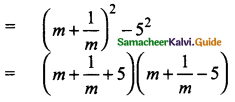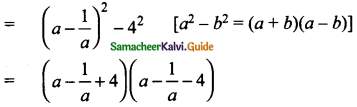Students can download Maths Chapter 3 Algebra Ex 3.5 Questions and Answers, Notes, Samacheer Kalvi 9th Maths Guide Pdf helps you to revise the complete Tamilnadu State Board New Syllabus, helps students complete homework assignments and to score high marks in board exams.

Tamilnadu Samacheer Kalvi 9th Maths Solutions Chapter 3 Algebra Ex 3.5

Question 1.
Factorise the following expressions:
(i) 2a² + 4a²b + 8a²c
(ii) ab – ac – mb + mc
Solution:
(i) 2a² + 4a²b + 8a²c = 2a²(1 + 2b + 4c)
(ii) ab – ac – mb + mc = a(b – c) – m(b – c)
= (b – c) (a – m)Question 2.
Factorise the following expressions:
(i) x² + 4x + 4
(ii) 3a² – 24ab + 48b²
(iii) x5 – 16x
(iv) m2 + $$\frac{1}{m^2}$$ – 23
(v) 6 – 216x2
(vi) a2 + $$\frac{1}{a^2}$$ – 18
Solution:
(i) x2 + 4x + 4 = x2 + 2 × x × 2 + 22 [a2 + 2ab+ b2 = (a + b)2]
= (x + 2)2

(ii) 3a2 – 24ab + 48b2 = 3[a2 – 8ab + 16b2]
= 3[a2 – 2 × a × 4b + (4b)2]
= 3(a- 4b)2 [a2 – 2ab + b2 = (a – b)2](iii) x5 – 16x = x[x4 – 16] [a2 – b2 = (a + b) (a – b)]
= x[(x2)2 – 42]
= x(x2 + 4) (x2 – 4)
= x(x2 + 4) (x2 – 22)
= x(x2+ 4) (x + 2) (x – 2)

(iv) m2 + $$\frac{1}{m^2}$$ – 23 = [Add + 2 and – 2 to make -23 as -25]
= m2 + $$\frac{1}{m^2}$$ + 2 – 2 – 23 = m2 + $$\frac{1}{m^2}$$ + 2 – 25
= m2 + $$\frac{1}{m^2}$$ + 2 × m × $$\frac{1}{m}$$ – 52(v) 6 – 216x2 = 6[1 – 36x2]
= 6[1 – (6x)2] [a2 – b2 = (a + b)(a – b)]
= 6(1 + 6x) (1 – 6x)

(vi) a2 + $$\frac{1}{a^2}$$ – 18 = a2 + $$\frac{1}{a^2}$$ – 2 + 2 – 18
(add -2 and +2 to make 18 as 16)
= a2 + $$\frac{1}{a^2}$$ – 2 × a × $$\frac{1}{a}$$ – 16 [a2 + b2 – 2ab = (a-b)(a- b)2]Question 3.
Factorise the following expressions:
(i) 4x2 + 9y2 + 25z2 + 12xy + 30yz + 20xz
Solution:
[a2 + b2 + c2 + 2ab + 2bc + 2ac = (a + b + c)2]
= (2x)2 + (3y)2 + (5z)2 + 2(2x) (3y) + 2(3y) (5z) + 2(5z) (2x)
= (2x + 3y + 5z)2

(ii) 25x2 + 4y2 + 9z2 – 20xy + 12yz – 30xz
Solution:
= (5x)2 + (2y)2 + (3z)2 + 2(5x) (-2y) + 2(-2y) (- 3z) + 2(-3z) (5x)
= (5x – 2y – 3z)2Question 4.
Factorise the following expressions:
(i) 8x3 + 125y3
(ii) 27x3 – 8y3
(iii) a6 – 64
Solution:
(i) 8x3 + 125y3 = (2x)3 + (5y)3 [a3 + b3 = (a + b)(a2 – ab + b2)
= (2x + 5y) [(2x)2 – (2x) (5y) + (5y)2]
= (2x + 5y) (4x2 – 10xy + 25y2)

(ii) 27x3 – 8y3 = (3x)3 – (2y)3 [a3 – b3 = (a – b)(a2 + ab + b2)]
= (3x – 2y) [(3x)2 + (3x) (2y) + (2y)2]
= (3x – 2y) (9x2 + 6xy + Ay2)(iii) a6 – 64 = a6 – 26
= (a2)3 – (22)3 [a2 – b3 = (a- b) + (a2 + ab + b2)]
= (a2 – 22) [(a2)2 + (a2) (22) + (22)2]
= (a + 2) (a – 2) (a4 + 4a2 + 16)
= (a + 2) (a – 2) [(a2)2 + 42 + 8a2 – 4a2]
= (a + 2)(a- 2) [(a2 + 4)2 – (2a)2] {a2 – b2 = (a + b) (a – b)}
= (a + 2) (a – 2) [(a2 + 4 + 2a) (a2 + 4 – 2a)
= (a + 2) (a – 2) (a2 + 2a + 4) (a2 – 2a + 4)

Question 5.
Factorize the following
(i) x3 + 8y3 + 6xy – 1
(ii) l3 – 8m3 – 27n3 – 18lmn
Using the formula [a3 + b3 + c3 – 3abc] = (a + b + c) (a2 + b2 + c2 – ab – bc – ac)
Solution:
(i) x3 + 8y2 + 6xy – 1 = -(-x3 – 8y3 – 6xy + 1)
= – (-x3 – 8y3 + 1 – 6xy)
= -[(-x)3 + (-2y)3 + 1 – 3(x) (2y) (1)]
= -[-x – 2y + 1] [(-x)2 + (-2y)2 + 12 – (-x) (-2y) – (-2y) (1) – (1) (-x)]
= (x + 2y – 1)(x2 + 4y2 + 1 – 2xy + 2y + x)(ii) l3 – 8m3 – 27n3 – 18lmn
= l3 + (-2m)3 + (-3n)2 – 3(l) (-2m) (-3n)
= (l – 2m – 3n) [l2 + (-2m)2 + (-3n)2 -1 (-2m)] – (-2m)(-3n) – (-3n)(l)
= (l – 2m – 3n) (l2 + 4m2 + 9n2 + 2lm – 6mn + 3ln)